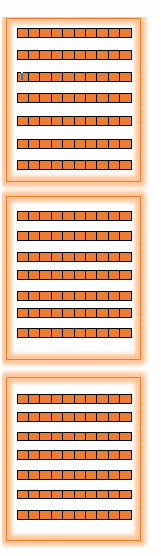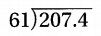Refer to our Texas Go Math Grade 5 Answer Key Pdf to score good marks in the exams. Test yourself by practicing the problems from Texas Go Math Grade 5 Module 4 Assessment Answer Key.

Concepts and Skills

Question 1.
Explain how the position of the decimal point changes in a quotient as you divide a number by 10,100, and 1,000. (TEKS 5.3.G)
Move the decimal point to the left for as many places (steps) as there are zeros in the factor 10, 100, or 1000.
Explanation:

28 ÷ 10=2.8  — Move the decimal point one step to the left.
28÷ 100 = 0.28 –Move the decimal point two steps to the left. You need to write zeros in front of the number.
28 ÷ 1000 = 0.028 — Move the decimal point three steps to the left. You need to write zeros in front of the number.

Explain how you can use base-ten blocks to find 2.16 ÷ 3. (TEKS 5.3.F)
Explanation:

Step 1:
Use base-ten blocks to show 2.16.
Remember that a flat represents one, a tong represents one tenth and a small cube represent one hundredth.
Therefore, 2 ones, 1 tenths, and 6 hundredths.Step 2
Share the ones.
Share the ones equally 3 groups.
There will be 0 one share in each group and 2 ones Left over _
( Notice that 2 ones cannot be shared among 3 groups without regrouping.
Therefore, you have to regroup the ones by rep(acing them with tenths)
step 3
Share the tenths.
(Remember that 2 ones (left over and the one is equal’ to 10 tenths.)
So, now We have 21 tenths in total.
And there will be 7 tenths share in each group, 21÷ 3Step 4:
Share the hundredths.
Share the 6 hundredths equally among the 3 groups.
There will be 2 hundredths share in each group, 6 ÷3step 5
Therefore,
2.16 ÷3 = 0.72

Complete the pattern. (TEKS 5.3.G)Question 3.
223 ÷ 1 = __________
223 ÷ 10 = __________
223 ÷ 100 = __________
223 ÷ 1,000 = __________
223 ÷ 1 = 223
223 ÷ 10 = 22.3
223 ÷ 100 = 2.23
223 ÷ 1,000 = 0.223
Explanation:
Move the decimal point to the left for as many places (steps) as there are zeros in the factor 10, 100, or 1000.

61 ÷ 1 = __________
61 ÷ 10 = __________
61 ÷ 100 = __________
61 ÷ 1,000 = __________
61 ÷ 1 = 61
61 ÷ 10 =6.1
61 ÷ 100 = 0.61
61 ÷ 1,000 = 0.061
Explanation:
Move the decimal point to the left for as many places (steps) as there are zeros in the factor 10, 100, or 1000.

Question 5.
57.4 ÷ 1 = __________
57.4 ÷ 10 = __________
57.4 ÷ 100 = __________
57.4 ÷ 1 = 57.4
57.4 ÷ 10 =5.74
57.4 ÷ 100 = 0.574
Explanation:
Move the decimal point to the left for as many places (steps) as there are zeros in the factor 10, 100, or 1000.

Estimate the quotient (TEKS 5.3.A)

Question 6.
31.9 ÷ 4
Explanation:
4 goes into 31, 7 times with remainder of 3
4 goes into 39, 9 times with remainder of 3
4 goes into 30, 7 times with remainder of 2
4 goes into 20, 5 times with no remainder 0

Question 7.
6.1 ÷ 8
Explanation:
8 goes into 61, 7 times with remainder of 5
8 goes into 50, 6 times with remainder of 2
8 goes into 20, 2 times with remainder of 4
8 goes into 20, 5 times with no remainder 0

492.6 ÷ 48
Explanation:
48 goes into 492, 10 times with remainder of 12
48 goes into 126, 2 times with remainder of 30
48 goes into 300, 6 times with remainder of 12
48 goes into 120, 2 times with no remainder 24
48 goes into 240, 5 times with no remainder 0

Use models or strategies to divide. (TEKS 5.3.F, 5.3.G)

Question 9.Explanation:
STEP 1
Use base-ten blocks to show 4.35.
Remember that a flat represents one. A long represents
One tenth, and a small cube represents one hundredth.
There are 4 one(S), 3 tenth(s), and 5 hundredth(s).
STEP 2 Share the ones.
4 one(S) cannot be shared among 5 groups without regrouping. Regroup the one(s) by replacing them with tenths.
There is 0 one(s) shared in each group and 4 one(s) left over.
There are now 43 tenth(s). And 8 tenth(s) shared in each group and 3 tenth(s).left over.
STEP 3 share the tenths.
Two tenths cannot be shared among 3 groups without regrouping. Regroup the tenths by replacing them with hundredths.
There are 0 tenth(s) shared in each group and 3 tenth(s) left over.
STEP 4 Share the hundredths.
Share the hundredths equally among the 5 groups.
There are  35 hundredth(s) and 7 hundredth(s) shared in each group and 0 hundredth(s) left ove
Question 10.Explanation:
9.92÷8=1.24
Done by base ten blocks method

Question 11.Explanation:
207÷61 = 3.4
Done by base ten blocks method

The Westside Bakery uses 440 pounds of sugar to make 1,000 cakes. Each cake contains the same amount of sugar. How many pounds of sugar does the bakery need if they bake only 100 cakes? (TEKS 5.3.G)
(A) 4.4 pounds
(B) 0.44 pound
(C) 0.044 pound
(D) 44 pounds
Explanation:
The Westside Bakery uses 440 pounds of sugar to make 1,000 cakes
440 ÷ 1000= 0.44
Each bread contains 0 . 44  pounds of sugar
To make 100 cakes
0.44 x 100 = 44 poundsQuestion 13.
Fourteen boxes of candles were sold for $91.00. Each box contains 5 candles. What is the cost of 1 candle? (TEKS 5.3.G) (A)$1.30
(B) $6.50 (C)$13.00
(D) $0.65 Answer: A Explanation: Fourteen boxes of candles were sold for$91.00
91 ÷ 14 = 6.5, so, each box cost is $6 . 5 Each box contains 5 candles 6.5 ÷ 5 = 1.30 so, each candle cost is$1. 30.

Question 14.
Jason has a piece of wire that is 62.4 inches long. He cuts the wire into 3 equal pieces. Which is the best estimate of the length of each piece of wire? (TEKS 5.3.A)
(A) 30 inches
(B) 3 inches
(C) 20 inches
(D) 2 inches
Explanation:
STEP 1 Use base-ten blocks to show 62.4.
Remember that a flat represents one. A long represents
One tenth, and a small cube represents one hundredth.
There are 6 one(S), 2 tenth(s), and 4 hundredth(s).
STEP 2 Share the ones.
Share an equal number of ones among 3 groups.
There is 2 one(s) shared in each group and 0 one(s) left over.
STEP 3 share the tenths. Two tenths cannot be shared among 3 groups without regrouping. Regroup the tenths by replacing them with hundredths.
There are 0 tenth(s) shared in each group and 2 tenth(s) left over.
There are now 24 hundredth(s).
STEP 4 Share the hundredths.
Share the 24 hundredths equally among the 3 groups.
There are 24 hundredth(s) and 8 hundredth(s) shared in each group and 0 hundredth(s) left over.
So, each piece of wire is 20.8 yards long.